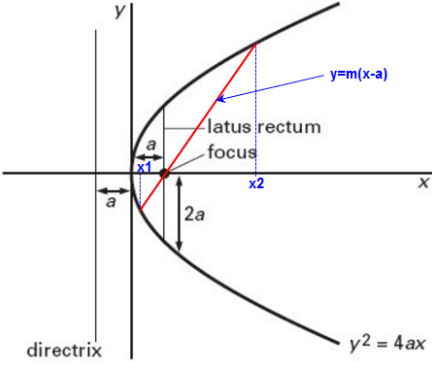#### If focus of a parabola is (2, 0) and one extremity of latus return is (2,2), then its equation is Option 1) y2= 4(3-x) Option 2) y2 = 4x - 4 Option 3) both (a) and (b) Option 4) none of theseStandard equation of parabola -- whereinOption 1)

y2= 4(3-x)

This solution is incorrect

Option 2)

y2 = 4x - 4

This solution is incorrect

Option 3)

both (a) and (b)

This solution is correct

Option 4)

none of these

This solution is incorrect